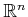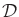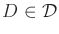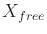#### Decomposinginto cells

At the outset,is decomposed into a collection of cells without considering collision detection. Suppose thatis an-dimensional rectangular subset of. Ifis more generally a smooth manifold, then the rectangular subset can be defined in a coordinate neighborhood. If desired, identifications can be used to respect the topology of; however, coordinate changes are technically needed at the boundaries to properly express velocities (recall Section 8.3).

The most common cell decomposition is obtained by splittinginto-dimensional cubes of equal size by quantizing each coordinate. This will be called a cubical partition. Assume in general thatis partitioned into a collectionof-dimensional cells. Letdenote a cell, which is a subset of. It is assumed here that all cells have dimension. In the case of cubes, this means that points on common boundaries between cubes are declared to belong to only one neighboring cube (thus, the cells may be open, closed, or neither).

Note thatis partitioned into cells, and not, as might be expected from the methods in Chapter 6. This means that collision detection and other constraints onare ignored when defining. The cells are defined in advance, just as grids were declared in Section 5.4.2. In the case of a cubical partition, the cells are immediately known upon quantization of each coordinate axis.

Steven M LaValle 2020-08-14